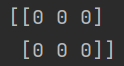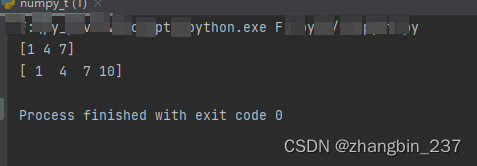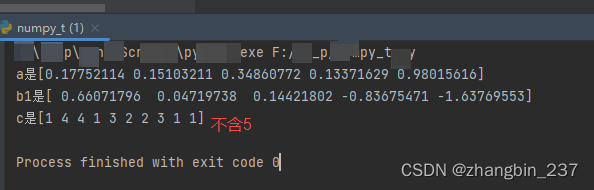• python创建类的数组
2020-11-29 01:45:15一、直接定义法：

1.直接定义matrix=[0,1,2,3]

2.间接定义matrix=[0 for i in range(4)]

print(matrix)

二、Numpy方法：

Numpy内置了从头开始创建数组的函数：zeros(shape)将创建一个用指定形状用0填充的数组。默认的dtype是float64。

下面是几种常用的创建方法：#coding=utf-8

import numpy as np

a = np.array([1,2,3,4,5])

print a

b = np.zeros((2,3))

print b

c = np.arange(10)

print c

d = np.arange(2,10,dtype=np.float)

print d

e = np.linspace(1.0,4.0,6)

print e

f = np.indices((3,3))

print f

三、其他转换法：

数组还有比较常用的一种方法，就是从其他Python结构（例如，列表，元组）转换。

下面给出一些例子。

列表转数组：a = []

a.append((1,2,4))

a.append((2,3,4))

a = np.array(a)

a.flatten()

元组转成数组：import numpy as np

mylist = [1,2,3]

print tuple(mylist)

iarray = np.array(tuple(mylist))

print iarray

更多相关内容
• 本文实例讲述了python创建关联数组（字典）的方法。分享给大家供大家参考。具体分析如下： 关联数组在python中叫字典，非常有用，下面是定义字典的两种方法 # Dictionary with quoted or variable keys d1 = {name:...
• 广告关闭腾讯云11.11云上盛惠 ，精选热门产品助力上云，云服务器首年88元起，买的越多返的越多，最高返5000元！java创建二维数组我门来看看java创建二... 创建一个python二位数组的尴尬也想模仿一下java，奈何只能：...广告关闭

腾讯云11.11云上盛惠 ，精选热门产品助力上云，云服务器首年88元起，买的越多返的越多，最高返5000元！java创建二维数组我门来看看java创建二维数组的格式：type arrayname; type arrayname; 比如：int arr=new int ; 一个5行3列的整数二维数组就创建了。 轻松写意... 创建一个python二位数组的尴尬也想模仿一下java，奈何只能：>>> li = file ,line 1 li = ^syntaxerror: invalid syntax抱歉，行不通。 但是可以这样，>...

npose = 5nsmile = 2 posesmile_cell = np.empty((npose,nsmile),dtype=object) for i in range(5): for k in range(2):posesmile_cell = np.zeros((4,4)) print posesmile_cell.shape参考文献：https:stackoverflow.comquestions19797822creating-matlab-cell-arrays-in-python...

python创建多维数组的3种方式：#coding=utf-8import numpy as np#1image = for row in range(3)]a =np.array(image)print(a) #2new_image =np.zeros((3,5)) #3b =np.arange(12).reshape(3,4)print(b)...

pylistobject 是一个变长对象，所以列表的长度是随着元素多少动态改变的。 同时它还支持插入和删除等操作，所以它还是一个可变对象。 可以简单理解为，python 的列表是长度可变的数组。 一般而已，我们用于列表创建都是一维数组。 那么问题来，我们如果创建多维数组呢？ 01 列表能创建多维数组？ 列表是支持操作符...

有谁知道如何在python中创建列表数组？ 例如，如果我希望最终结构包含第一个维度固定但第二个维度不同的数据： 指数0：指数1： 指数2...

numpy（numeric python，以numpy导入）是一系列高效的、可并行的、执行高性能数值运算的函数的接口。 numpy模块提供了一种新的python数据结构——数组(array)，以及特定于该结构的函数工具箱。 该模块还支持随机数、数据聚合、线性代数和傅里叶变换等非常实用的数值计算工具。 下面将学习如何创建不同形状的numpy数组...

所以我有一个python从各种daq单元收集数据点，我想要做的就是将它们吐出成csv。 我的代码与我的示例数组完美配合，但我现在无法让它在我的生活中工作。 所以这就是我所拥有的： with open(filename, w) as output:writer = csv.writer(output, delimiter = ,) writer.writerow(header) i = 0totalruns = numtest...

给定一个包含n个元素的数组，需要生成包含k行的后续数组： , , , ... ]. 如何有效地获得这个？...

我有一个数组a，我想在字典中存储每5个值，同时以编程方式创建键。 例如： 如果我们有 a= 我希望字典看起来像这样： d={first_title:, second_title:}...我们之前学习了手动建立和利用特殊函数numpy.zeros以及numpy.ones来创建数组。 这节课我们提供更多的方法，让大家可以创建自己想要的数组。 01从其他数据类型转换我们在讲列表和numpy数组很像的时候，告诉大家一种讲列表转换成numpy数组的方法。 其实numpy里面有一个函数，叫asarray可以不仅可以将列表转换成numpy...

数组如果我们需要一个只包含数字的列表，那么array.array比list更高效。 数组支持所有跟可变序列有关的操作,包括.pop,.insert和.extend。 另外，数组还提供从文件读取和存入文件的更快的方法，如.frombytes和.tofile。 python数组跟c语言数组一样精简。 创建数组需要一个类型码，这个类型码用来表示在底层的c语言应该...

注：每一步可能依赖于上一步import的模块 一、数组1,用numpy对数组进行运算from numpy importarraymm=array((1, 1, 1))pp=array((1, 2, 3))则pp+mm 输出 array()pp*2 array()pp**2 array()2,访问数组元素pp输出23,多维数组jj = array(, ])注：上面这句话的意思是创建下面这样一个多维数组jj =? 获取该数组元素：jj 第...

python没有提供内置的数组类型，但是通过标准库array提供了array类支持数组的创建与使用，可以创建的数组类型包括整数、实数、unicode字符等等，可以使用help()函数查看更完整的类型列表。 from array import array>>> s = hello world>>> sa = array(u, s)#创建可变字符串对象>>> print(sa)array(u, hello world)>>>...

initializer：初始化器，若数组为空，则省略初始化器。 arr = array.array(i,)print(arr)#array.typecodes——模块属性print(n输出一条 包含所有可用类型代码的字符串：print(array.typecodes)#注意，调用者是模块名，不是某个对象#array.typecode——对象属性print(n输出 用于创建数组的类型代码字符：print(arr...

题目意思很简单，就是将数组往后移动 k 个位置，超出数组长度的从头开始计算。 如果只是这个要求，题目特别简单，新开一个数组，然后将原数组移动 k 保留到对应位置即可。 而题目的难点在于需要“原地”移动，空间复杂度为 o(1)，即不能新创建数组。 参考答案 首先分析题意，很容易想出移动位置公式：target_pos = ...

python中的列表(list)类似于c#中的可变数组（arraylist），用于顺序存储结构。 创建列表 sample_list = python 列表操作 sample_list = 得到列表中的某一个值value_start = sample_list end_value = sample_list删除列表的第一个值 delsample_list在列表中插入一个值 sample_list = 得到列表的长度 list_length =...作为示例，我们先在python中创建一个二维的numpy数组, 并写入二进制文件：import numpy as np>>> a = np.array(range(100),dtype = np.float32)>>> b = a.reshape((4,-1))>>>barray(, , , ], dtype=float32)>>>b.tofile(d:numpydata.ha)接着在c++中从该文件读取数据，放入二维数组中，并将每个元素加1，然后将改变后...

我正在尝试根据我的商店创建新的文件_数组列表如果目录中还不存在名称，则创建一个新的，然后创建另一个。 我需要创建300个文件。 store_array = if store_number == 1:continue print(store_number, file=open(rc:usershankdesktopprojectjson_ + +json, w)) typeerror：必须是str，而不是list我可以通过一个简单的...

获取 python 版本的方法：linux shell\$python -vpython 2. 7.11windows cmdd:> python -vpython 2. 7.11如果提示不是内部或者外部命令， 请先在 window 环境变量 path 里面添加上 python 的绝对路径。 生成客户端对象secretid=xxxxxx #替换为用户的 secretidsecretkey = xxxxxx#替换为用户的 secretkeyendpoint = ...整体代码如下：#-*- coding:utf-8 -*-#stacking.pyimport numpy as np #创建数组a = np.arange(9).reshape(3,3)print(a)#out:#array(,# ,# ]) b = 2 * a ...#array(,# ,# ,# ,# ,# ], dtype=bool)小结今天学习一下python中numpy的堆叠数组。 希望通过上面的操作能帮助大家。 如果你有什么好的意见，建议，或者有...

展开全文• ## python创建多维数组

千次阅读 2022-04-12 22:15:05
python多维数组的建立

目录

一、使用numpy

二、基本语法构建

推荐使用numpy

### 一、使用numpy

import numpy as np

ls = np.zeros((2,3),int)当然除了numpy的zeros函数，还有ones, arrage等函数

### 二、基本语法构建

#一维
a =  * 9

#二维
#二维数组
demo = [ * 9 for _ in range(0, 3)]

#三维
#三维数组
demo = [[ * 9 for _ in range(0, 3)] for _ in range(0, 3)]

注意需要反过来建立

展开全文python 多维数组
• python numpy创建数组

numpy是python的第三方包，使用时需要引入包

1、等差数组

基本格式 arange(a,b,c)，其中a、b、c都为数字，等差数组的范围为a（含）到b（不含）；

c如果忽略不设置，默认为1；

如果只设置一个参数，为0到a的、差为1的等差数组

import numpy as np

a=np.arange(1,10,3)
b=np.arange(1,11,3)
print(a)
print(b)


执行结果：2、随机数组

基本格式：

random.rand()                            -----[0,1)的随机数

random.randn()                          -----均值为0，标准差为1的正态分布的随机数数组

random.randint()                        -----指定范围内、指定个数的整数数组（范围含左不含右）

import numpy as np

a=np.random.rand(5)
b1=np.random.randn(5)
c=np.random.randint(1,5,10)
print('a是'+str(a))
print('b1是'+str(b1))
print('c是'+str(c))


执行结果：展开全文python 机器学习
• # 这样的话创建出的其实是3*3*?的数组，最后一维是动态的 # 如果希望最后一维是固定长度的话，把参数中的0替换成想要的长度也就可以了，这样的话是用0填充了 方法2：手撸函数def high_dim_list(shape, fix_last = ...pytorch 数组
• Numpy内置了从头开始创建数组的函数： zeros(shape)将创建一个用指定形状用0填充的数组。默认的dtype是float64。 下面是几种常用的创建方法： ''' 学习中遇到问题没人解答？小编创建了一个Python学习交流QQ群：...python
• ## 从Python列表创建数组

千次阅读 热门讨论 2021-11-10 20:19:46python
• Numpy内置了从头开始创建数组的函数：zeros(shape)将创建一个用指定形状用0填充的数组。默认的dtype是float64。 下面是几种常用的创建方法： #coding=utf-8 import numpy as np a = np.array([1,2,3,4,5]) ...python 机器学习
• 上面一段代码创建了一个空二维数组，但 [item]*n这种方法简单的调用了item的默认复制方法 上面item是一个数组，因此对任意row[i]修改都会影响所有row[j]。 正确的做法是用for循环 row = [[] for _ in range(9)] ...
• import numpy as np #自定义数据类型 dt = np.dtype([('name','S20'),('age','i1'),('score','f4')]) #自定义数组 u=np.array([('abc',12,88),('...#创建数组 e=np.empty([3,4],dtype=int,order='C') print(e) .python
• ## python怎么创建数组

千次阅读 2020-12-11 04:35:40
间接定义matrix=[0 for i in range(4)]　print(matrix)二　Numpy方法：Numpy内置了从头开始创建数组的函数：zeros(shape)将创建一个用指定形状用0填充的数组。默认的dtype是float64。下面是几种常用的创建方法：#...
• ## Python3 创建数组

千次阅读 2020-05-28 11:22:39
创建2维数组 python3 Python 3.7.4 (default, Aug 13 2019, 20:35:49) [GCC 7.3.0] :: Anaconda, Inc. on linux Type "help", "copyright", "credits" or "license" for more information. >>> len =5 &...python
• 直接使用 “=” 复制，浅拷贝，两个数组地址一样，改变其中一个，另一个也变化 int[] a1 = {1, 2,3}; int[] a2 = a1; a1 = 0; System.out.println(Arrays.toString(a1)); //[0,2,3] System.out.println(Arrays....
• Python创建二维数组 Python中的列表list可以当做一维数组使用，但是没有直接的定义使用二维数组。如果直接使用a = [][]会产生SyntaxError: invalid syntax语法不正确错误。 一般Python创建二维数组的方法是使用...
• Python创建数组的三种方式： 1、numpy指定形状为0 实际上，empty生成的数组当然可以为空，只要我们指定了相应的形状。例如，如果我们传入数组的形状参数为（0，3），则可以生成目标空数组： 所以，生成的数组是否...python java
• 间接定义matrix=[0 for i in range(4)]print(matrix)二、Numpy方法：Numpy内置了从头开始创建数组的函数：zeros(shape)将创建一个用指定形状用0填充的数组。默认的dtype是float64。下面是几种常用的创建方法：#...
• python 数组添加数组Python doesn’t have any specific data type as an array. We can use List that has all the characteristics of an array. Python没有任何特定的数据类型作为数组。 我们可以使用具有数组...python 数据结构
• ## Python生成JSON数组

千次阅读 2021-06-02 15:43:41
自己找了很多，没找到数组的，最后还是看了好多网页，自己研究的 # - 20210602 LET import json json_txt = { 'code': rand_code, 'protocols': [] } json_data['data'] = json_txt #---- protocols 数组...python json
• 在工作示例程序（脚本）中，某些数据数组的定义如下：x_data = np.random.rand(100).astype(np.float32)当我随后在Python控制台中键入“x_data”时，它返回>>> x_dataarray([ 0.16771448, 0.55470788, 0.36438608, ....
•python 数据挖掘
• python里面已经没有了多维数组的概念，直接用的张量来表示多维数组，其实也就换了个名字 二、用法 1、一维数组 重复方法定义一维数组 numpy方法一维数组 二维数组 重复方法定义二维数组 numpy方法二维数组 ...python
• I'm preparing a data set to run in the program rpy (R, which runs in Python) for statistical analysis. It looks like this:data = [[0, 1, 0, 0, 0, 0, 0, 1, 0, 0], [0, 0, 1, 0, 0, 1, 0, 0, 0, 0],[0, 1, ...
• 方法1： #dp = [0 for _ in range(target+1)] #dp = 1 方法2： dp = +*target 方法2貌似比方法1块...